# python tutorial - Python String Format | String Formatting in Python using % - learn python - python programming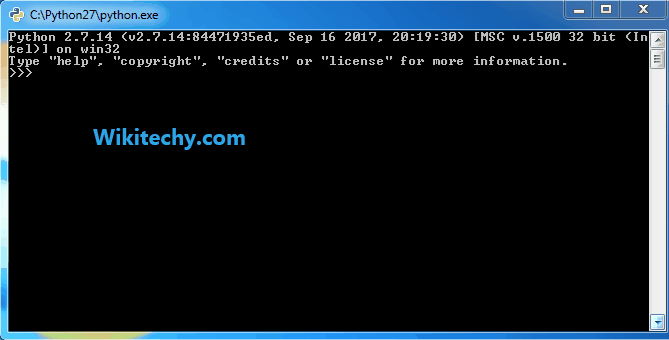Learn Python - Python tutorial - python string format - Python examples - Python programs

## Formatting Strings

• Two flavors of string formatting:
• string formatting expressions: this is based on the C type printf.
• string formatting method calls: this is newer and added since Python 2.6.

## % operator

• The % provides a compact way to code several string substitutions all at once.

## Format specification

Code Meaning
b Binary format. Outputs the number in base 2.
c Character. Converts the integer to the corresponding unicode character before printing.
d Decimal Integer. Outputs the number in base 10.
Default.
e, E Exponent notation. Prints the number in scientific notation using the letter e/E to indicate the exponent.
f, F Fixed point. Displays the number as a fixed-point number.
g

General format. For a given precision p >= 1, this rounds the number to p significant digits and then formats the result in either fixed-point format or in scientific notation, depending on its magnitude.
The precise rules are as follows: suppose that the result formatted with presentation type e and precision p-1 would have exponent exp. Then if -4 <= exp < p, the number is formatted with presentation type f and precision p-1-exp. Otherwise, the number is formatted with presentation type e and precision p-1. In both cases insignificant trailing zeros are removed from the significant, and the decimal point is also removed if there are no remaining digits following it.
Positive and negative infinity, positive and negative zero, and nans, are formatted as inf, -inf, 0, -0 and nan respectively, regardless of the precision.
A precision of 0 is treated as equivalent to a precision of 1.
Default.

G General format. Same as g except switches to E if the number gets too large. The representations of infinity and NaN are uppercased, too.
n Number. This is the same as g, except that it uses the current locale setting to insert the appropriate number separator characters.
o Octal format. Outputs the number in base 8.
s String format. This is the default type for strings and may be omitted.
x, X Hex format. Outputs the number in base 16, using lower/upper- case letters for the digits above 9.
% Percentage. Multiplies the number by 100 and displays in fixed (f) format, followed by a percent sign.

## Syntax format

• The example below is using left justification(-) and zero(0) fills.
• For floating point number, in the following example, we're using left justification, zero padding, numeric + signs, field width, and digits after the decimal point.
• We can simply convert them to string using a format expression or str built-in function:
• In case when the sizes are unknown until runtime, we can have the width and precision computed by specifying them with a * in the format string to force their values to be taken from the next item in the inputs to the right of the %. In the example, the 4 in the tuple gives precision:

In Python a string of required formatting can be achieved by different methods.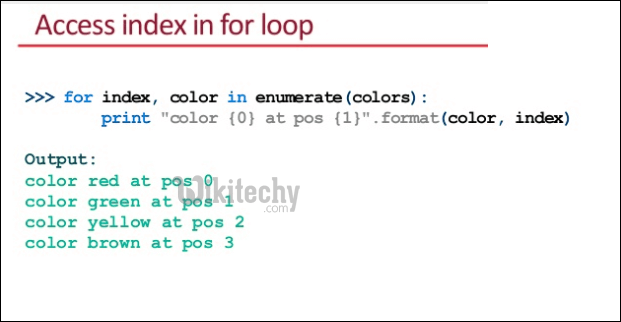Some of them are ;
1) Using %
2) Using {}
3) Using Template Strings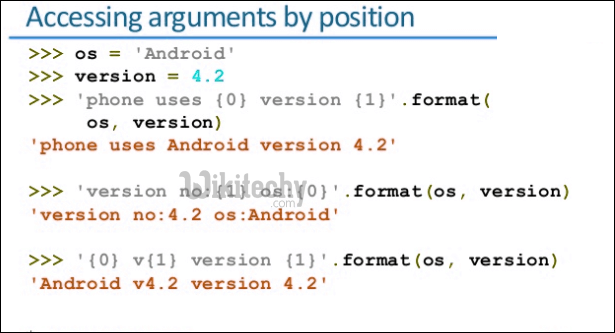The formatting using % is similar to that of ‘printf’ in C programming language.
%d – integer
%f – float
%s – string
%o – octal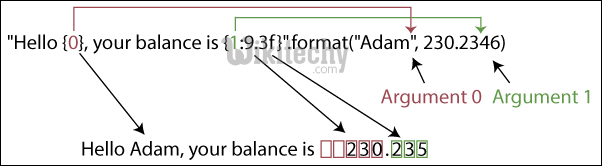The below example describes the use of formatting using % in Python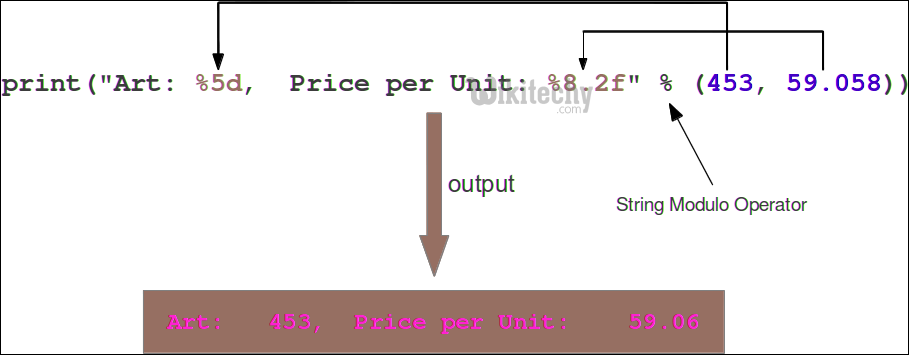python - Sample - python code :python programming - Output :

Wikitechy tutorial site provides you all the learn python , python programming help ,online python learning , learn to program with python , online python training free